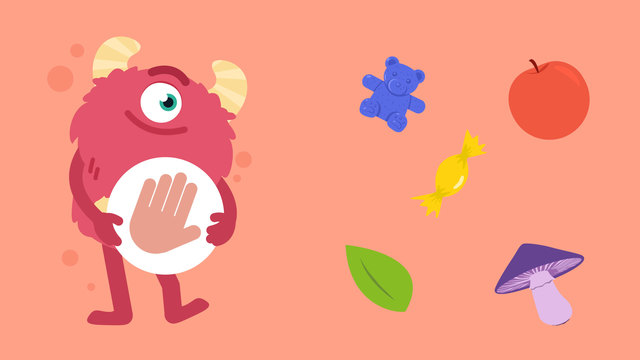# Sort and Count— Let's Practice!Rating

Ø 4.0 / 11 ratings
The authorsTeam Digital
Sort and Count— Let's Practice!
CCSS.MATH.CONTENT.K.MD.B.3

## Basics on the topicSort and Count— Let's Practice!

### TranscriptSort and Count— Let's Practice!

Razzi says get your fingers ready because today we're going to "Sort and Count." It's time to begin! Sort these objects into categories by color! Let's start by finding all the red apples. How many red apples are there? There are three of them. Did you also find three apples? Let's tackle the next problem! Now find all the yellow candies. How many yellow candies are there? There are two of them. Did you also find two yellow candies? Let's find some more! Find all the green leaves. How many green leaves are there? There are three of them. Did you also get three? Let's try another! Find all the BLUE bears. How many blue bears are there? There are four! Did you also get four? Let's practice one more time! Find all the purple mushrooms. How many purple mushrooms are there? There is only one! Did you also find one? Razzi had so much fun practicing with you today! See you next time!

1.111111111111111111111111111

From Dakota Jacobs, about 1 year ago
2.qqqqqqqqqqqqqqqqqqqqqqqqqqqqqqqqqqqqqqqqqqqqqqqqqqqqqqqqqqqqqqqqqqqqqqqqqqqqqqqqqqqqqqqqqqqqqqqqqqqqqqqqqqqqqqqqqqqqqqqqqqqqqqqqqqqqqqqqqqqqqqqqqqqqqqqqqqqqqqqqqqqqqqqqqqqqqqqqqqqqqqqqqqqqqqqqqqqqqqqqqqqqqqqqqqqqqqqqqqqqqqqqqqqqqqqqqqqqqqqqqqqqqqqqqqqqqqqqqqqqqqqqqqqqqqqqqqqqqqqqq

From Dakota Jacobs, about 1 year ago

## Sort and Count— Let's Practice! exercise

Would you like to apply the knowledge you’ve learned? You can review and practice it with the tasks for the video Sort and Count— Let's Practice!.
• ### How many of each object is there?

Hints

Here you can see the name of each fruit.

Choose one fruit and find all of them in the image. How many are there?

For example, if we were were finding out how many strawberries there are, we would find the strawberries and then count them. 1...2!

Solution

The totals are: Strawberries: 2

• We can see them circled in blue.
Bananas: 1
• We can see it circled in pink.
Apples: 4
• We can see them circled in purple.
Oranges: 3
• We can see them circled in black.

• ### Can you answer the questions?

Hints

First, count each shape. This is what they look like.

Compare the amounts.

• Which shape occurs the most?
• Which shape is there fewest of?

• If there are more, that means there is a greater number of that shape.
• If there are fewer, that means there is a smaller number of that shape.
Solution
1. There are 5 squares.
2. There are 6 circles.
3. There are 3 triangles.
4. I can see more circles than squares.
5. I can see fewer triangles than squares.
• ### Can you sort and count the vegetables?

Hints

These are the names of each vegetable.

Start by finding all of the carrots and writing how many there are. Then repeat this with the peas and onions.

Solution

Here we can see the completed table.

• ### Can you sort the items?

Hints

Here are the names of each item.

You could start by finding all of the candy and filling in the total then repeat this with the rest of the items.

Solution

Here are the totals.

• ### Can you find the total number of each object?

Hints

Look at each individual picture and then see how many of that object is in the picture at the top.

Here we can see all of the purple crayons circled, how many are there?

Solution
• There are 2 purple crayons (circled in pink).
• There is 1 pair of scissors (circled in blue).
• There are 4 orange cubes (circled in green).
• There is 1 book (circled in black).
• ### Can Lena make a cake?

Hints

Here you can see each ingredient labelled.

Count the ingredient and then compare the total to what is needed to bake the cake. Is it the same or is it lower?

Here we can see 3 bags of sugar circled. How many bags of sugar does Lena need? Is 3 enough?

Remember, write the word yes in the last box if there is enough. Write the word no if there is not enough.

Solution
• Lena has 3 bags of flour. Does she have enough? Yes
• Lena has 3 bags of sugar. Does she have enough? No, she needs one more bag to make 4.
• Lena has 8 packs of butter. Does she have enough? Yes
• Lena has 11 eggs. Does she have enough? No, she needs one more to make 12.